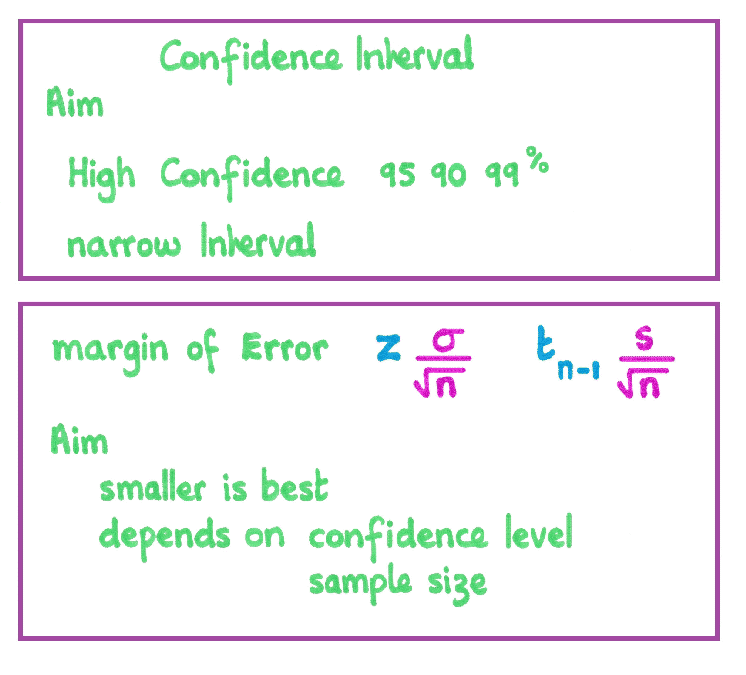Coming to Statistica
in 2015/2016

Group Classes

Interactive Webinars

Blog

Guestbook

.

E-mail        anne@statistica.com.au

# Place         Willetton, Western Australia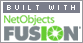# Mathematics & Statistic Tutor Perth - SPSS Help

This page will discuss general Confidence Interval Formation and concentrate on the Confidence Interval for the Population Mean.

Most Confidence Interval have a similar format

Sample Statistic plus / minus  (z or t)  multiplied by  appropriate Standard Error

# Point Estimate versus Interval Estimate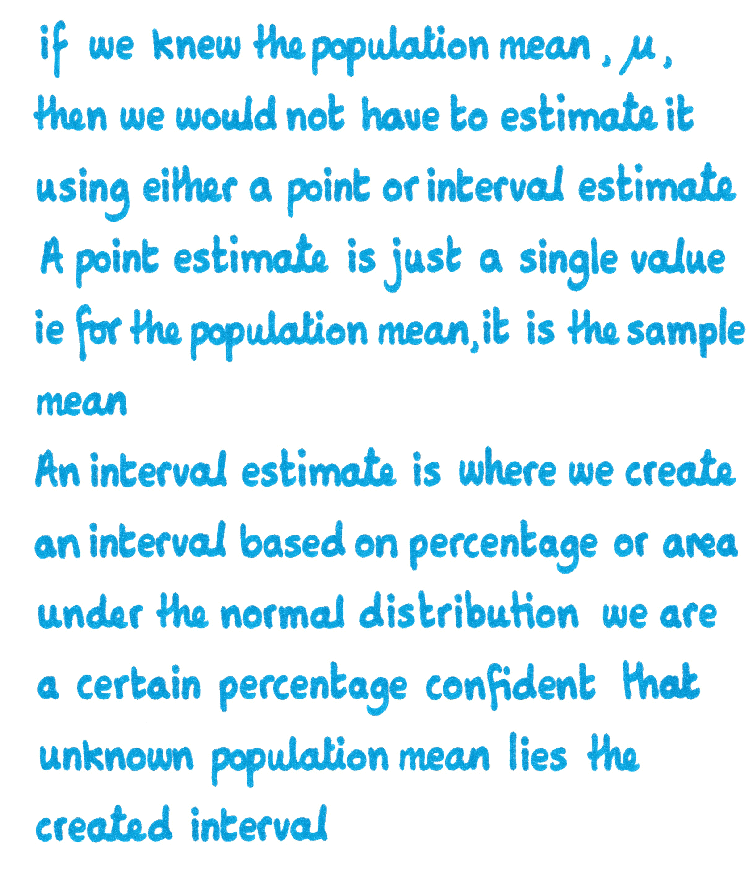Please make sure you understand that it is a confidence interval and not a probability as the true value of the population parameter either lies in the interval or not.

From the diagrams below, you will see the theory of how an interval is created by using the area underneath the curve and the symmetry of the curve.

The standardised curve is used so the centre value is Zero rather than the value of the Population Mean.

So these Z -values will be change depending on the area trapped but will always be the same value regardless of the value or nature of the Population parameter.

For example, if we wanted a 95% confidence interval of a population proportion then
Z-value would be 1.96 provided we had a normal distribution.

You will see that not all the intervals created will contain the true value of the population parameter (this example is based on the Population Mean though any measure may be used).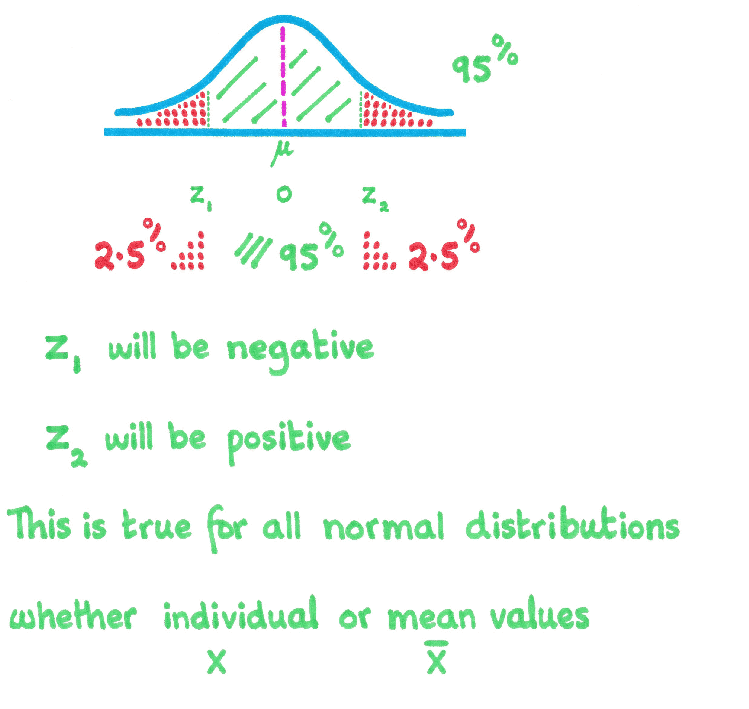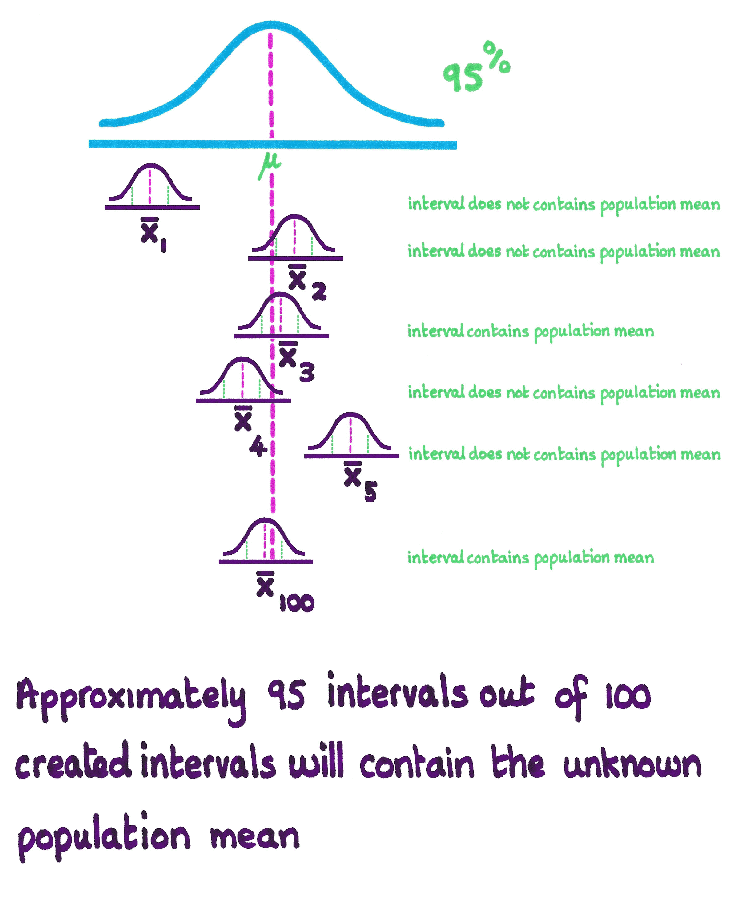Most Confident Intervals follow the pattern

Sample Statistic add / subtract  ( Margin of Error )

Margin of Error  is (z or t value) multiplied  appropriate Standard Error

Remember to check whether it is Population or Sample Standard Deviation as this may affect whether it is z or t is used in formula.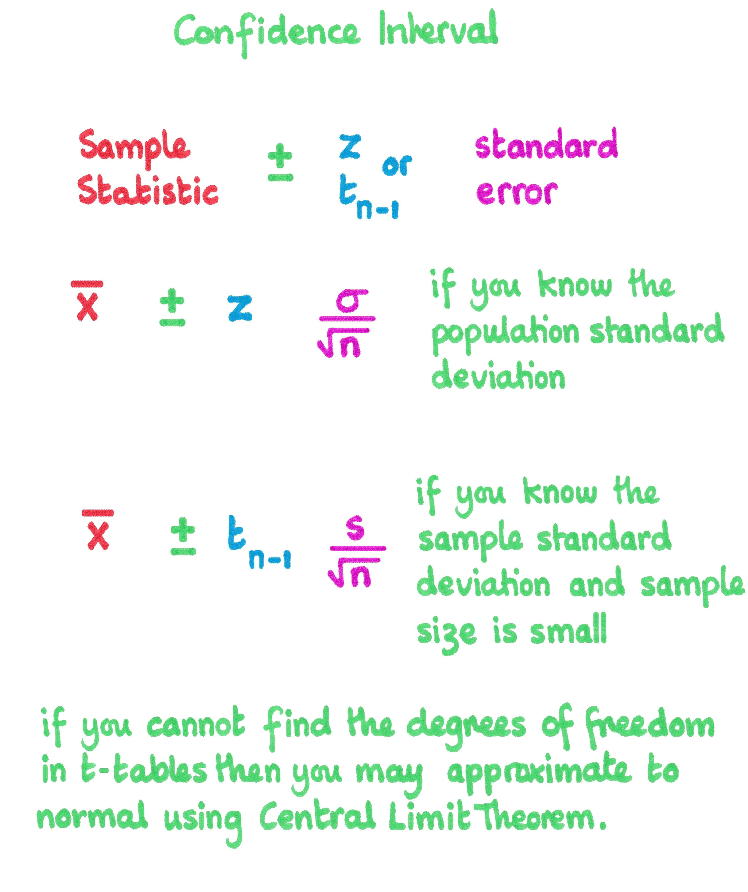Confident Interval facts

It is an interval so you have an Upper and Lower limit.

You can use the Margin of Error to calculate the minimum sample size needed at a certain level of confidence.

The notation  Z 0.05 , this indicates that this is the Z value has an area of 0.05 on the right hand side of the value. The z-value is 1.645

If you are using a t-value and not sure which column to use, the last line contains the      z-values.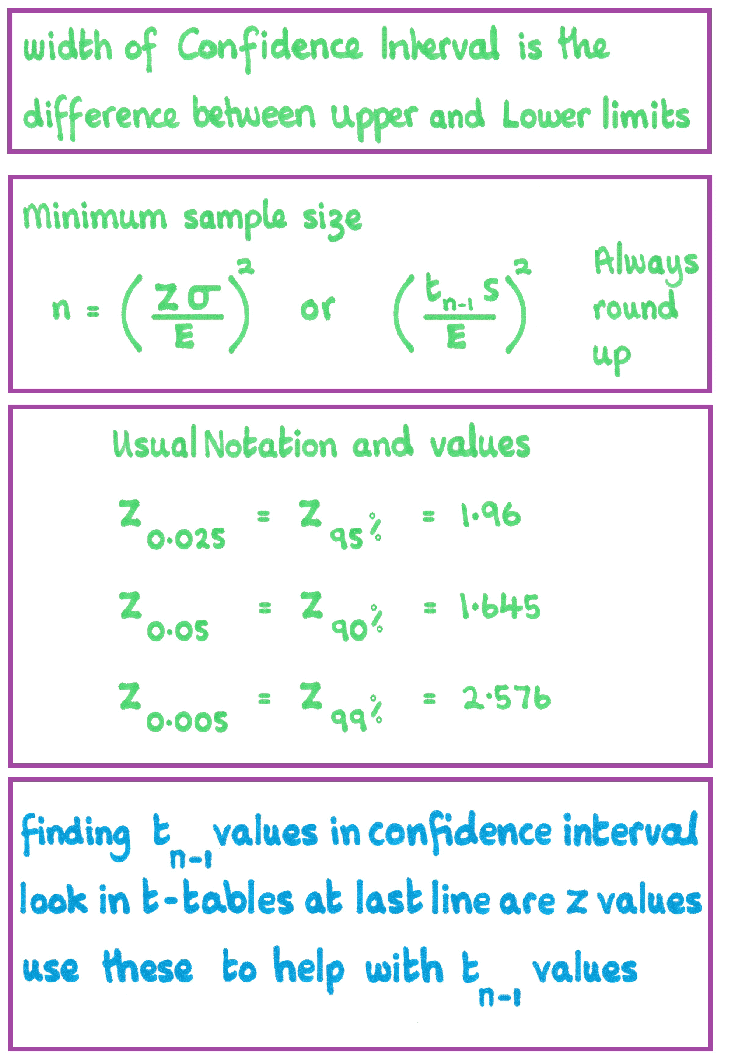What makes a good Confident Interval
and
how can we reduce the Margin of Error